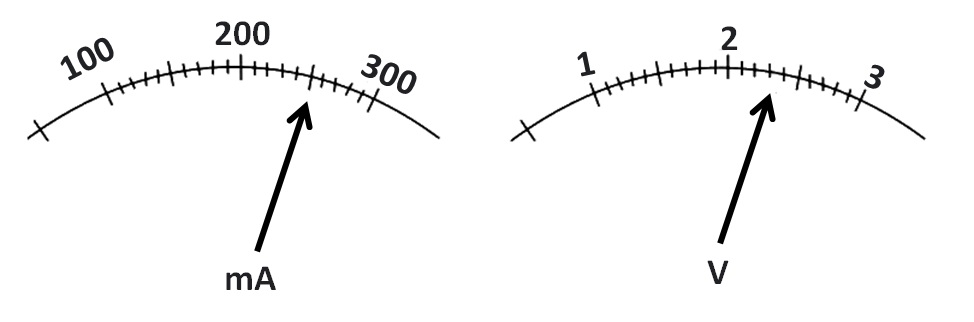Past Year - 2 Mark Questions

Class 10
Chapter 12 Class 10 - Electricity

## (b) What is the resistance of the resistor?(a)

 Ammeter Voltmeter For Ammeter,  We see that 10 Divisions is equal to 100 Milli Amperes   So,  Least Count = 100/10 mA = 10 mA = 10 × 10 -3 A = 10 -2 A = 0.01 A For Voltmeter,  We see that 10 Divisions is equal to 1 Volt   So,  Least Count = 1/10 = 0.1 V

(b)

According to ohm’s law,

Resistance = V / I

= 2.4 Volts / 250 mA

= 2.4 Volts / 250 * 10 -3 A

= 2.4 Volts / 0.25 A

= 9.6 ohm

Learn in your speed, with individual attention - Teachoo Maths 1-on-1 Class